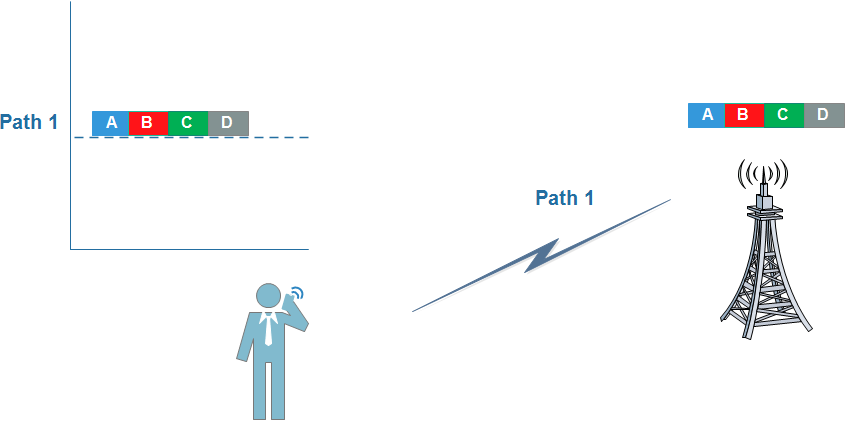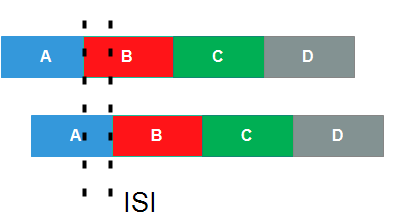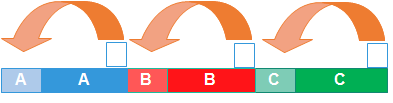# What is Inter Symbol Interference (ISI) in LTE, How Cyclic Prefix (CP) helps eliminating this problem ?#### What is Inter Symbol Interference (ISI) ?

In an ideal system (theoretical), the transmitted symbols arrive at the receiver without any loss or interference, as shown in the following figure.But in a real scenario, due to the signal propagation phenomena, such as reflection, diffraction or multipath, a receiver can receive several delayed versions of the same signal.At the receiver, all these “multipath” components are summed. And the practical result is that we have multiple symbols being received “simultaneously” (Symbols “Overlap”) – this is the inter symbol interference (ISI)!

And when symbols partially overlap with each other and information present at the edges may get lost.

Due to multipath,  the same information is received at multiple instance of time. The total delay in first copy of some information and the last received copy of the same signal is called delay spread. So the distortion in the symbol reaches upto delay spread.If the symbol period (T) is very short compared to the “Delay Spread” (t) the impact is significant (T << t). But if we can extend the symbols length, most of them will not suffer the impact of ISI (T >> t). One small part of the symbol will continue to be impacted, but for most of its duration, the symbol will remain not affected by reflections propagated in “Multipath”.

#### What is physical meaning of CP (Cyclic Prefix) ?

The cyclic prefix acts as a buffer region or guard interval to protect the OFDM signals from inter symbol interference.

We have learned that we minimize the ISI a lot when we make the size of the symbol larger (the size of the delay spread becomes relatively minor compared to the larger size). But as much as we could increase this symbol size, the effects of ISI would always be present.### How CP helps

To permanently eliminate this problem, the solution is to find a way where the ‘lost’ part of the symbol could be ‘recovered’.

The one way to do this is by copying or duplicating an initial part of the symbol, and inserting the end of it. And it acts as Cyclic Prefix.So here we understands that the CP copy a small part of the initial information (hence the name prefix) to the end of each symbol (hence the name cyclic).

Thus, the receiver can identify the end points of each symbol and correctly correlate the information, thereby eliminating the interference problem.

In LTE, two types of CPs are defined. Normal and Extended.

The normal cyclic prefix is intended to be sufficient for the majority of scenarios, while the extended cyclic prefix is intended for scenarios with particularly high delay spread.